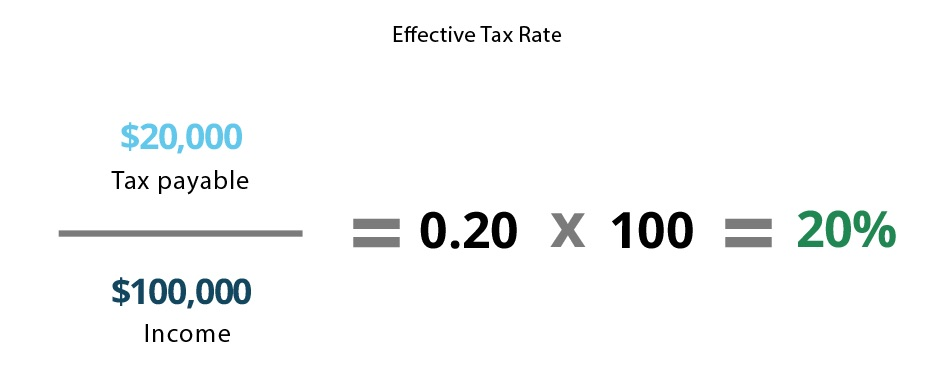# Marginal Tax Rate

Reviewed by:
ImageWritten by:
ImageUpdated August 22, 2020

## What Is Marginal Tax Rate?

Marginal tax rate is the rate at which an additional dollar of taxable income would be taxed. It is part of a progressive tax system, which applies different tax rates to different levels of income. As income rises, it is taxed at a higher rate (according to the bracket it falls in).

It’s important to note that income is not taxed at a single rate, but at multiple rates according to each tax bracket it falls in. Each tax rate only applies to income that falls within the corresponding bracket, and each additional dollar beyond that bracket would be taxed at the next highest marginal rate.

## Examples of Marginal Tax Rate

Sarah is an accountant for XYZ firm and has a taxable income of \$100,000 per year. Here’s how her income would be taxed using sample marginal tax rates:

Tax bracketMarginal Tax RateAmount taxableTax payable
\$0-\$20,0000%\$20,000\$0
\$20,000-\$40,00010%\$20,000\$2,000
\$40,000-\$60,00020%\$20,000\$4,000
\$60,000-\$80,00030%\$20,000\$6,000
\$80,000-\$100,00040%\$20,000\$8,000
\$100,000+50%\$0\$0
Total\$100,000\$20,000

As you can see, Sarah’s income is taxed at different rates as it moves through the tax schedule, increasing as it enters each new bracket. Since any additional earnings would fall into the \$100,000+ bracket, her marginal tax rate would be 50%.

## How to Calculate Marginal Tax Rate

Marginal tax rate is calculated by multiplying the income in a given bracket by the adjacent tax rate.

When considering how marginal tax rate will affect an increase in income, you must first consider where in the bracket your current income lies. If your income will still remain in the same bracket after the increase, you’d simply multiply the increase by the corresponding tax rate. Here’s an example:

John’s taxable income is \$50,000 per year, meaning his marginal tax rate is 20%. He gets a raise of \$10,000, which brings his income to \$60,000 per year. The additional income would be taxed at 20% because that income still falls within his current tax bracket. In this situation, his raise would be subject to: \$10,000 x 0.20 = \$2,000 in taxes.

Tax bracketTax Rate
\$0-\$20,0000%
\$20,000-\$40,00010%
\$40,000-\$60,00020%
\$60,000-\$80,00030%

If an increase in income would push your income into the next tax bracket, the increase would be subject to two tax rates; it would be taxed at your current marginal tax rate until it reaches the top end of your current bracket, and any amount that exceeds your current bracket would be taxed at the next highest rate. Here’s an example:

John’s income is \$50,000 per year, meaning his marginal tax rate is 20%. John gets a raise of \$15,000, bringing his income to \$65,000 per year. The first \$10,000 of his raise would be taxed at 20% because it’s within his current bracket, but the next \$5,000 would be taxed at 30% because it falls within the next bracket. In this situation, his raise would be subject to: (\$10,000 x 0.20 = \$2,000) +  (\$5,000 x 0.30 = \$1,500) = \$3,500 in taxes

Tax bracketTax Rate
\$0-\$20,0000%
\$20,000-\$40,00010%
\$40,000-\$60,00020%
\$60,000-\$80,00030%

## Marginal Tax Rate Common Misconceptions

Filing your taxes can be a daunting task. Not only are there all sorts of terms and forms to be aware of, but there are also a variety of different tax rates that are easily confused. Here’s how marginal tax rate compares to other common tax rates:

### Marginal vs. Effective Tax Rate

Unlike marginal tax rate – which is the highest rate that applies to your income – an effective tax rate is the overall percentage of your income that goes towards taxes. It’s calculated by dividing the total amount of tax payable by pre-tax income.

Using the example above, Sarah’s effective tax rate would be:In this situation, Sarah’s effective tax rate would be 20% (compared to her marginal tax rate of 50%).

### Marginal Tax Rate vs. Flat Tax Rate

A flat tax rate is exactly what it sounds like: It’s a single tax rate that is applied to all incomes, regardless of the amount. Using Sarah’s \$100,000 income example, a flat tax of 15% means that Sarah would owe \$15,000 in taxes.

It’s worth noting that some states utilize a flat income tax rate, such as Colorado, Illinois, Indiana, Massachusetts, Michigan, New Hampshire, Pennsylvania, and Tennessee.

## 2020 US Federal Income Tax Brackets

Marginal tax rates and brackets can change from year to year, like the adjustments made by the Tax Cuts and Jobs Act in 2018. While rates and income brackets could be subject to change in the future, here are the 2020 US Federal Income Tax Brackets:

Tax rate

Single filer

Income above

Married individuals filing jointly

Income above

Married individuals filing separately

Income above